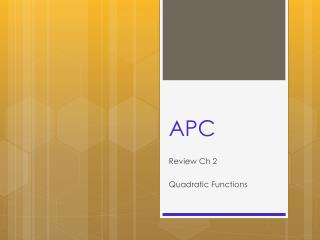DownloadDownload PresentationAPC

# APC

Download Presentation## APC

- - - - - - - - - - - - - - - - - - - - - - - - - - - E N D - - - - - - - - - - - - - - - - - - - - - - - - - - -
##### Presentation Transcript

1. APC Review Ch 2 Quadratic Functions

2. Quiz on Thursday 2.1-2.6 • Function notation • Domain and Range • Minimum and maximums • Even and odd functions • Library of functions • Piece-wise functions • Transformations

3. Function notation

4. Graph f(x) = x4 -3x3 +4x -1 • Find all local maximum and minimums • State the intervals on which the function is increasing • State the intervals on which the function is decreasing

5. Test for even and odd functions

6. Sketch each of the functions from the library of functions • Sketch it • Name it • State the Domain and Range • State even, odd, or neither

7. Average Rate of Change

8. Sketch this graph (1) horizontally stretched by a factor or 2 and vertically shifted up 3

9. Quadratic Functions • What is old? f(x) = ax2 + bx + c • Find the vertex • Find the x intercepts • What is new? f(x) = a(x-h)2 +k • Where (h, k) is the vertex • Graph using transformations

10. Changing from general to vertex form • Completing the square with lead coefficient not 1 • Sheet #4